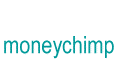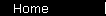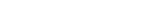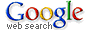The calculator on this site lets you put the basic financial formulas to work, with more flexibility than the interactive graphs on these pages.

The Rule of 72 shows how to estimate compound interest in your head.

The article on Monte Carlo retirement planning starts out with a simple retirement calculator (compound interest and contributions going up, annuity coming down) and then lets you add randomness to simulate the volatility of real investments.

The stock valuation article has several calculators based on discounted earnings and related rules of thumb.

The CAGR of the Stock Market discusses average versus annualized returns (a.k.a. arithmetic versus geometric means) and shows how they're related via standard deviation.

Two useful books on investment valuation formulas:

John Burr Williams' Theory of Investment Value is an old classic, with lots of equations as well as lots of commonsense advice (the kind people relearn during every bear market and then forget again a couple of years later);

Damodaran's Investment Valuation is the modern reference you see on the desk of all "quants" everywhere.

home  |  article  |  glossary  |  calculator  |  about us  |  booksIntroductionCompound InterestMore CompoundingPresent Value / CAGRComposite InvestmentsBond YieldGeometric SeriesGrowth + ContributionsAnnuityMortgageStock ValuationBooks & Links